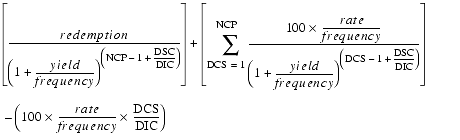# PRICE

Description Computes the price of a security with specified rate, redemption value, and yield.

Syntax PRICE ( settlement, maturity, rate, yield, redemption, frequency [, calendar_type] )
Argument Description
settlement The date when the security is traded to the buyer. Decimal values will be truncated to integers.
maturity The date the security expires and the remaining amount is paid to the investor. It must be later than settlement. Decimal values will be truncated to integers.
rate The security's annual coupon rate. The coupons pay at this rate divided by frequency.
yield The annual income produced by the security.
redemption The security's redemption value per \$100 face value. This is the amount paid at the settlement date that is not part of any final coupon payment.
frequency The number of interest payments per year. See The frequency Argument for more information.
[calendar_type] Optional. One of five methods of counting days for computing interest. See The calendar_type Argument for more information.

Remarks Use this function when all coupon periods between settlement and maturity are of equal length. If the first coupon period is shorter or longer than the rest, use ODDFPRICE. If the last coupon period is shorter or longer than the rest, use ODDLPRICE.

Equationwhere the three-letter codes correspond to values you can compute using the coupon functions, as shown in the following table.
Code Meaning Function
DCS Number of days from beginning of the coupon period to settlement. COUPDAYBS
DIC Number of days in coupon period in which settlement falls. COUPDAYS
DSC Number of days from settlement to the next coupon. COUPDAYSNC
NCP Number of coupons payable between settlement and redemption. COUPNUM

Examples This function returns 90.35:

`PRICE("4/19/97","11/25/01",0.05,0.075,100,4)`